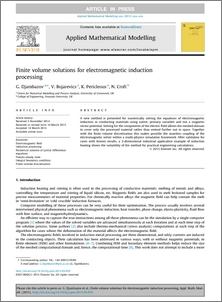# Finite volume solutions for electromagnetic induction processing

, , and Croft, N. (2015) Finite volume solutions for electromagnetic induction processing. Applied Mathematical Modelling, 39 (16). pp. 4733-4745. ISSN 0307-904X (doi:https://doi.org/10.1016/j.apm.2015.03.059)Preview
PDF (Publisher's In Press Version [Gold OA - CC BY])
13588_PERICLEOUS_Finite_Volume_Solutions_2015.pdf - Published Version

## Abstract

A new method is presented for numerically solving the equations of electromagnetic induction in conducting materials using native, primary variables and not a magnetic vector potential. Solving for the components of the electric field allows the meshed domain to cover only the processed material rather than extend further out in space. Together with the finite volume discretisation this makes possible the seamless coupling of the electromagnetic solver within a multi-physics simulation framework. After validation for cases with known results, a 3-dimensional industrial application example of induction heating shows the suitability of the method for practical engineering calculations

Item Type: Article Electromagnetic field; Induction processing; Numerical solution of partial differential equations; Pseudo-steady state; Integral boundary condition; Finite volume discretisation Q Science > QA Mathematics > QA75 Electronic computers. Computer science Faculty of Engineering & Science > Centre for Numerical Modelling & Process Analysis (CNMPA) > Computational Science & Engineering Group (CSEG)Faculty of Engineering & Science > School of Computing & Mathematical Sciences (CMS)Faculty of Engineering & Science 04 Mar 2022 13:07 http://gala.gre.ac.uk/id/eprint/13588

### Actions (login required)View Item